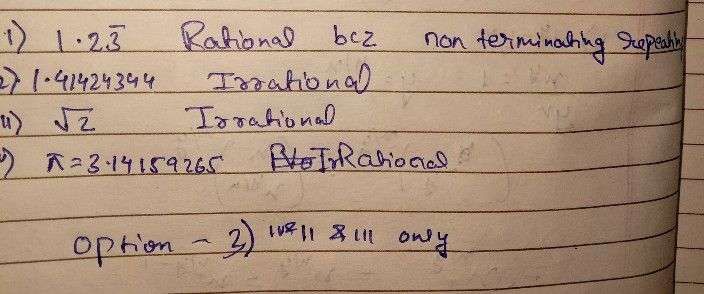Symbol
ProblemWhich of the following is irrational $i\right)$ $1.2\bar{3}$ $i1\right)$ $1.41424344$ $.4$ ( $...$ $iii\right)$ $\sqrt{2}$ $iV\right)$ $\pi$ $1\right)$ i and iv $2\right)$ ii and iii only $3\right)$ ii, iii and iv $4\right)$ i only
10th-13th grade
Other
SolutionQanda teacher - kavita❤️Student
thanks mam. By the way you are so hotQanda teacher - kavita❤️
?Student
I love you
you are from